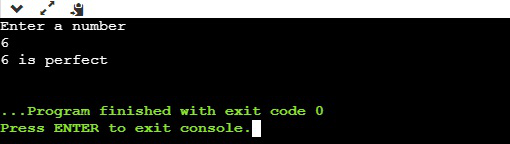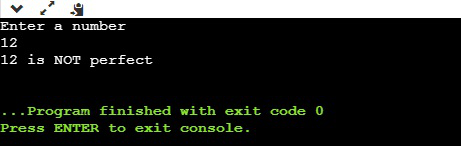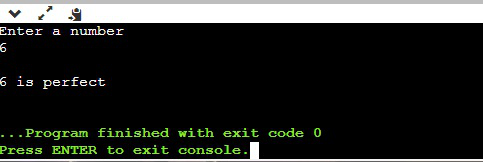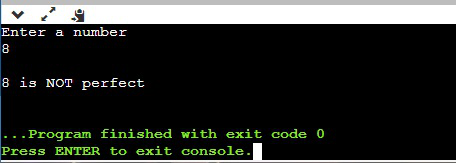# Bash shell script to find if a number is perfect or not

• Last Updated : 23 Aug, 2021

In this article, we will discuss how to write a bash script to find if a number is perfect or not. A perfect number is defined as, a positive number that is equal to the sum of its proper divisors. Smallest no is 6 ex= 1,2,3 are divisor of 6 and 1+2+3=6

## Method 1: Using While Loop

• Then run while loop with condition i <= no/2 .
• Check if no%i is equals to 0 or not if true then add i to ans .
• After getting out of the while loop checks if the obtained value of variable sum is equal to no variable or not.
• If equals then return “\$no is a perfect number ” else “\$no is not a perfect number ” using echo command.
```# !/bin/bash

echo "Enter a number"

# initializing the value of i
i=1

ans=0

# check if the value of left operand is less
# than or equal to the value of right operand
# if yes, then the condition becomes true
while [ \$i -le \$((no / 2)) ]

do
# Checks if the value of two operands are
# equal or not; if yes, then the condition
# becomes true
if [[ \$((no%i)) -eq 0  ]]
then

ans=\$((ans + i))

fi

i = \$((i + 1))

done

# Checks if the value of two operands are equal
# or not; if yes, then the condition becomes true
if [ \$no -eq \$ans ]
then
# printing output
echo "\$no is perfect"
else

# printing output
echo "\$no is NOT perfect"
fi```

Output:

1) perfect numberFig= output 1

2) not a perfect numberFig= output 2

## Method 2: Using For Loop

• Then use for loop and iterate until no(input).
• Check if no%i is equaled to 0 or not if true then add i to ans .
• After getting out of for loop check if the obtained value of the variable sum is equal to no variable or not .
• If equals then return “\$no is a perfect number ” else “\$no is not a perfect number ” using echo command.
```# !/bin/bash
echo "Enter a number"
i=1
ans=0
for i in 1 2 3 4 5 .. no
do
if [[ \$((no%i)) -eq 0  ]]
then
ans=\$((ans + i))

fi
i=`expr \$i + 1`
done

if [ \$no -eq \$ans ]
then
echo "\$no is perfect"
else
echo "\$no is NOT perfect"
fi```

Output:

1) perfect numberFig= perfect

2) not perfect numberFig=not perfect

My Personal Notes arrow_drop_up